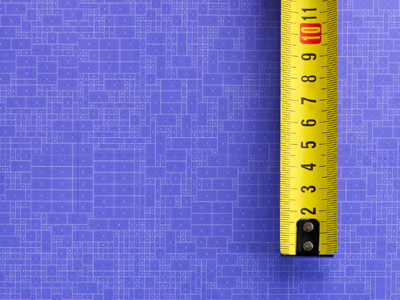There are 10 millimeters in a centimeter.

# Decimal Numbers 2

This Math quiz is called 'Decimal Numbers 2' and it has been written by teachers to help you if you are studying the subject at elementary school. Playing educational quizzes is an enjoyable way to learn if you are in the 3rd, 4th or 5th grade - aged 8 to 11.

It costs only \$12.50 per month to play this quiz and over 3,500 others that help you with your school work. You can subscribe on the page at Join Us

Decimal numbers are those that are smaller than a unit and are written after a decimal point. Decimal numbers are an alternative way of writing fractions. A quarter of a pizza written as a fraction would be 14, but written as a decimal would be 0.25. 0.25 is a decimal with two places - the 2 represents 2 tenths and the 5 is 5 hundredths.

Can you tell the difference between tenths and hundredths? See how well you understand decimal numbers by playing this quiz.

1.
Which is the smallest of these numbers?
0.12
1.02
0.02
0.01
1100 is less than 2100, 12100 and 102100
2.
Which is the decimal fraction equivalent to seven and forty two hundredths?
7.042
7.42
0.742
74.2
Forty two hundredths is equivalent to four tenths and two hundredths
3.
What does the digit 2 represent in 5.12?
2 tens
2 tenths
2 units
2 hundredths
In decimals the first column after the units is tenths and the second column is hundredths
4.
Which is the largest?
0.35
0.05
0.03
0.3
35100 is larger than 30100, 5100 or 3100
5.
How is 3.57 changed to 3.6?
Subtract 0.3
Subtract 0.03
Just like 357 + 3 = 360
3.57 + 0.03 = 3.6
6.
How is 0.07 changed to 0.02?
Subtract 0.5
Subtract 0.05
Just like 7 - 5 = 2
0.07 - 0.05 = 0.02
7.
What is 8.5cm in mm?
8.5mm
85mm
850mm
8,500mm
There are 10mm in 1cm therefore we multiply by 10 to find the answer
8.
What is the next number in the following sequence? 0.24, 0.26, 0.28, 0.3
0.32
0.35
1.3
1.32
the numbers are going up by 0.02, or 2100 every time
9.
What is the figure 6 worth in 0.26?
6 hundredths
6 units
6 tenths
6 tens
Remember - tenths, hundredths, thoudandths
10.
Which is a decimal fraction halfway between 2.1 and 2.2?
2.12
2.13
2.14
2.15
There are ten hundredths in a tenth so half of one tenth is 5 hundredths
Author:  Amanda Swift